Theory and Modern Applications

# Approximation of a generalized additive mapping in multi-Banach modules and isomorphisms in multi-${C}^{\ast }$-algebras: a fixed-point approach

## Abstract

Let $\mathcal{X}$, $\mathcal{Y}$ be vector spaces. It is shown that if an odd mapping $f:\mathcal{X}\to \mathcal{Y}$ satisfies the functional equation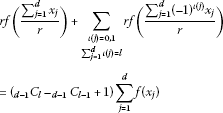(0.1)

then the odd mapping $f:\mathcal{X}\to \mathcal{Y}$ is additive, and we use a fixed-point method to prove the Hyers-Ulam stability of the functional equation (0.1) in multi-Banach modules over a unital multi-${C}^{\ast }$-algebra. As an application, we show that every almost linear bijection $h:\mathcal{A}\to \mathcal{B}$ of a unital multi-${C}^{\ast }$-algebra $\mathcal{A}$ onto a unital multi-${C}^{\ast }$-algebra $\mathcal{B}$ is a ${C}^{\ast }$-algebra isomorphism when $h\left(\frac{{2}^{n}}{{r}^{n}}uy\right)=h\left(\frac{{2}^{n}}{{r}^{n}}u\right)h\left(y\right)$ for all unitaries $u\in U\left(\mathcal{A}\right)$, all $y\in \mathcal{A}$, and $n=0,1,2,\dots$ .

MSC:39B52, 46L05, 47H10, 47B48.

## 1 Introduction

Throughout this paper we assume that r is a positive rational number and d, l are integers with $1.

Let X and Y be Banach spaces. Consider a mapping $f:X\to Y$ such that $f\left(tx\right)$ is continuous in $t\in \mathbb{R}$ for each fixed $x\in X$, and assume that there exist constants $\theta \ge 0$ and $p\in \left[0,1\right)$ with

$\parallel f\left(x+y\right)-f\left(x\right)-f\left(y\right)\parallel \le \theta \left({\parallel x\parallel }^{p}+{\parallel y\parallel }^{p}\right),\phantom{\rule{1em}{0ex}}x,y\in X.$

Rassias  showed that there exists a unique $\mathbb{R}$-linear mapping $T:X\to Y$ such that

$\parallel f\left(x\right)-T\left(x\right)\parallel \le \frac{2\theta }{2-{2}^{p}}{\parallel x\parallel }^{p},\phantom{\rule{1em}{0ex}}x\in X.$

Găvruta  extended the above theorem as follows: let G be an Abelian group, Y be a Banach space and put

$\stackrel{˜}{\phi }\left(x,y\right)=\sum _{j=0}^{\mathrm{\infty }}\frac{1}{{2}^{j}}\phi \left({2}^{j}x,{2}^{j}y\right)<\mathrm{\infty },\phantom{\rule{1em}{0ex}}x,y\in G.$

If $f:G\to Y$ is a mapping satisfying

$\parallel f\left(x+y\right)-f\left(x\right)-f\left(y\right)\parallel \le \phi \left(x,y\right),\phantom{\rule{1em}{0ex}}x,y\in G,$

then there exists a unique additive mapping $T:G\to Y$ such that

$\parallel f\left(x\right)-T\left(x\right)\parallel \le \frac{1}{2}\stackrel{˜}{\phi }\left(x,x\right),\phantom{\rule{1em}{0ex}}x\in G.$

Park  applied Găvruta’s result to linear functional equations in Banach modules over a ${C}^{\ast }$-algebra. Several functional equations have been investigated in [4, 5] and . In 2006 Baak, Boo and Rassias  solved the following functional equation: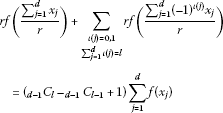(1.1)

(any solution of (1.1) will be called a generalized additive mapping) and proved its Hyers-Ulam stability in Banach modules over a unital ${C}^{\ast }$-algebra via the direct method. These results were applied to investigate ${C}^{\ast }$-algebra isomorphisms in unital ${C}^{\ast }$-algebras.

In this paper, we prove the Hyers-Ulam stability of the functional equation (1.1) in multi-Banach modules over a unital multi-${C}^{\ast }$-algebra via the fixed-point method. These results are applied to investigate ${C}^{\ast }$-algebra isomorphisms in unital multi-${C}^{\ast }$-algebras.

## 2 Fixed-point theorems

We recall two fundamental results in the fixed-point theory.

Theorem 2.1 [8, 9]

Let $\left(X,d\right)$ be a complete metric space and let $J:X\to X$ be strictly contractive, i.e.,

$d\left(Jx,Jy\right)\le Ld\left(x,y\right),\phantom{\rule{1em}{0ex}}x,y\in X$

for a Lipschitz constant $L<1$. Then

1. (1)

the mapping J has a unique fixed point ${x}^{\ast }\in X$,

2. (2)

the fixed point ${x}^{\ast }$ is globally attractive, i.e.,

$\underset{n\to \mathrm{\infty }}{lim}{J}^{n}x={x}^{\ast },\phantom{\rule{1em}{0ex}}x\in X,$
3. (3)

the following inequalities hold:

$\begin{array}{c}d\left({J}^{n}x,{x}^{\ast }\right)\le {L}^{n}d\left(x,{x}^{\ast }\right),\hfill \\ d\left({J}^{n}x,{x}^{\ast }\right)\le \frac{1}{1-L}d\left({J}^{n}x,{J}^{n+1}x\right),\hfill \\ d\left(x,{x}^{\ast }\right)\le \frac{1}{1-L}d\left(x,Jx\right)\hfill \end{array}$

for all $x\in X$ and nonnegative integers n.

Let X be a non-empty set. A function $d:X×X\to \left[0,\mathrm{\infty }\right]$ is called a generalized metric on X if for any $x,y,z\in X$, we have:

1. (1)

$d\left(x,y\right)=0$ if and only if $x=y$,

2. (2)

$d\left(x,y\right)=d\left(y,x\right)$,

3. (3)

$d\left(x,z\right)\le d\left(x,y\right)+d\left(y,z\right)$.

Theorem 2.2 [8, 10]

Let $\left(X,d\right)$ be a complete generalized metric space and let $J:X\to X$ be a strictly contractive mapping with a Lipschitz constant $L<1$. Then, for each $x\in X$, either

$d\left({J}^{n}x,{J}^{n+1}x\right)=\mathrm{\infty },\phantom{\rule{1em}{0ex}}n\ge 0$

or there exists a positive integer ${n}_{0}$ such that:

1. (1)

$d\left({J}^{n}x,{J}^{n+1}x\right)<\mathrm{\infty }$, $n\ge {n}_{0}$,

2. (2)

the sequence $\left({J}^{n}x\right)$ converges to a fixed point ${y}^{\ast }$ of J,

3. (3)

${y}^{\ast }$ is the unique fixed point of J in the set $Y=\left\{y\in X:d\left({J}^{{n}_{0}}x,y\right)<\mathrm{\infty }\right\}$,

4. (4)

$d\left(y,{y}^{\ast }\right)\le \frac{1}{1-L}d\left(y,Jy\right)$, $y\in Y$.

## 3 Multi-normed spaces

The notion of a multi-normed space was introduced by Dales and Polyakov . This concept is somewhat similar to an operator sequence space and has some connections with operator spaces and Banach lattices. Motivations for the study of multi-normed spaces and many examples are given in .

Let $\left(\mathcal{E},\parallel \cdot \parallel \right)$ be a complex normed space and $k\in \mathbb{N}$. We denote by ${\mathcal{E}}^{k}$ the linear space $\mathcal{E}\oplus \cdots \oplus \mathcal{E}$ consisting of k-tuples $\left({x}_{1},\dots ,{x}_{k}\right)$, where ${x}_{1},\dots ,{x}_{k}\in \mathcal{E}$. The linear operations on ${\mathcal{E}}^{k}$ are defined coordinate-wise. The zero element of either $\mathcal{E}$ or ${\mathcal{E}}^{k}$ is denoted by 0. Finally, we denote by ${\mathbb{N}}_{k}$ the set $\left\{1,\dots ,k\right\}$ and by ${\mathrm{\Sigma }}_{k}$ the group of permutations on k symbols.

Definition 3.1 [11, 14] A multi-norm on $\left\{{\mathcal{E}}^{k}:k\in \mathbb{N}\right\}$ is a sequence

$\left({\parallel \cdot \parallel }_{k}\right)=\left({\parallel \cdot \parallel }_{k}:k\in \mathbb{N}\right)$

such that ${\parallel \cdot \parallel }_{k}$ is a norm on ${\mathcal{E}}^{k}$ for $k\in \mathbb{N}$, ${\parallel \cdot \parallel }_{1}=\parallel \cdot \parallel$, and for any integer $k\ge 2$, we have

(A1) ${\parallel \left({x}_{\sigma \left(1\right)},\dots ,{x}_{\sigma \left(k\right)}\right)\parallel }_{k}={\parallel \left({x}_{1},\dots ,{x}_{k}\right)\parallel }_{k}$, $\sigma \in {\mathrm{\Sigma }}_{k}$, ${x}_{1},\dots ,{x}_{k}\in \mathcal{E}$,

(A2) ${\parallel \left({\alpha }_{1}{x}_{1},\dots ,{\alpha }_{k}{x}_{k}\right)\parallel }_{k}\le \left({max}_{i\in {\mathbb{N}}_{k}}|{\alpha }_{i}|\right){\parallel \left({x}_{1},\dots ,{x}_{k}\right)\parallel }_{k}$, ${\alpha }_{1},\dots ,{\alpha }_{k}\in \mathbb{C}$, ${x}_{1},\dots ,{x}_{k}\in \mathcal{E}$,

(A3) ${\parallel \left({x}_{1},\dots ,{x}_{k-1},0\right)\parallel }_{k}={\parallel \left({x}_{1},\dots ,{x}_{k-1}\right)\parallel }_{k-1}$, ${x}_{1},\dots ,{x}_{k-1}\in \mathcal{E}$,

(A4) ${\parallel \left({x}_{1},\dots ,{x}_{k-1},{x}_{k-1}\right)\parallel }_{k}={\parallel \left({x}_{1},\dots ,{x}_{k-1}\right)\parallel }_{k-1}$, ${x}_{1},\dots ,{x}_{k-1}\in \mathcal{E}$.

A sequence $\left(\left({\mathcal{E}}^{k},{\parallel \cdot \parallel }_{k}\right):k\in \mathbb{N}\right)$ is then said to be a multi-normed space.

Lemma 3.2 [11, 13]

Suppose that $\left(\left({\mathcal{E}}^{k},{\parallel \cdot \parallel }_{k}\right):k\in \mathbb{N}\right)$ is a multi-normed space. Then for any $k\in \mathbb{N}$, we have

1. (a)

${\parallel \left(x,\dots ,x\right)\parallel }_{k}=\parallel x\parallel$, $x\in \mathcal{E}$,

2. (b)

${max}_{i\in {\mathbb{N}}_{k}}\parallel {x}_{i}\parallel \le {\parallel \left({x}_{1},\dots ,{x}_{k}\right)\parallel }_{k}\le {\sum }_{i=1}^{k}\parallel {x}_{i}\parallel \le k{max}_{i\in {\mathbb{N}}_{k}}\parallel {x}_{i}\parallel$, ${x}_{1},\dots ,{x}_{k}\in \mathcal{E}$.

From Lemma 3.2(b), it follows that if $\left(\mathcal{E},\parallel \cdot \parallel \right)$ is a Banach space, then $\left({\mathcal{E}}^{k},{\parallel \cdot \parallel }_{k}\right)$ is a Banach space for each $k\in \mathbb{N}$ (in this case we say that $\left(\left({\mathcal{E}}^{k},{\parallel \cdot \parallel }_{k}\right):k\in \mathbb{N}\right)$ is a multi-Banach space).

Now, we recall two important examples of multi-norms (see [11, 12]).

Example 3.3 The sequence $\left({\parallel \cdot \parallel }_{k}:k\in \mathbb{N}\right)$ on $\left\{{\mathcal{E}}^{k}:k\in \mathbb{N}\right\}$ defined by

${\parallel \left({x}_{1},\dots ,{x}_{k}\right)\parallel }_{k}:=\underset{i\in {\mathbb{N}}_{k}}{max}\parallel {x}_{i}\parallel ,\phantom{\rule{1em}{0ex}}{x}_{1},\dots ,{x}_{k}\in \mathcal{E}$

is a multi-norm called the minimum multi-norm. The terminology ‘minimum’ is justified by property (b) from Lemma 3.2.

Example 3.4 Let $\left\{\left({\parallel \cdot \parallel }_{k}^{\alpha }:k\in \mathbb{N}\right):\alpha \in A\right\}$ be a (non-empty) family of all multi-norms on $\left\{{\mathcal{E}}^{k}:k\in \mathbb{N}\right\}$. For $k\in \mathbb{N}$, set

$⦀\left({x}_{1},\dots ,{x}_{k}\right){⦀}_{k}:=\underset{\alpha \in A}{sup}{\parallel \left({x}_{1},\dots ,{x}_{k}\right)\parallel }_{k}^{\alpha },\phantom{\rule{1em}{0ex}}{x}_{1},\dots ,{x}_{k}\in \mathcal{E}.$

Then $\left(⦀\cdot {⦀}_{k}:k\in \mathbb{N}\right)$ is a multi-norm on $\left\{{\mathcal{E}}^{k}:k\in \mathbb{N}\right\}$ called the maximum multi-norm.

Lemma 3.5 

Suppose that $k\in \mathbb{N}$ and $\left({x}_{1},\dots ,{x}_{k}\right)\in {\mathcal{E}}^{k}$. For each $j\in \left\{1,\dots ,k\right\}$, let ${\left({x}_{n}^{j}\right)}_{n\in \mathbb{N}}$ be a sequence in $\mathcal{E}$ such that ${lim}_{n\to \mathrm{\infty }}{x}_{n}^{j}={x}_{j}$. Then for each $\left({y}_{1},\dots ,{y}_{k}\right)\in {\mathcal{E}}^{k}$ we have

$\underset{n\to \mathrm{\infty }}{lim}\left({x}_{n}^{1}-{y}_{1},\dots ,{x}_{n}^{k}-{y}_{k}\right)=\left({x}_{1}-{y}_{1},\dots ,{x}_{k}-{y}_{k}\right).$

Definition 3.6 [12, 14]

Let $\left(\left({\mathcal{E}}^{k},{\parallel \cdot \parallel }_{k}\right):k\in \mathbb{N}\right)$ be a multi-normed space. A sequence ${\left({x}_{n}\right)}_{n\in \mathbb{N}}$ in $\mathcal{E}$ is said to be a multi-null sequence if for each $\epsilon >0$, there exists an ${n}_{0}\in \mathbb{N}$ such that

$\underset{k\in \mathbb{N}}{sup}{\parallel \left({x}_{n},\dots ,{x}_{n+k-1}\right)\parallel }_{k}<\epsilon ,\phantom{\rule{1em}{0ex}}n\ge {n}_{0}.$

We say that the sequence ${\left({x}_{n}\right)}_{n\in \mathbb{N}}$ is multi-convergent to $x\in \mathcal{E}$ and write ${lim}_{n\to \mathrm{\infty }}{x}_{n}=x$ if ${\left({x}_{n}-x\right)}_{n\in \mathbb{N}}$ is a multi-null sequence.

Definition 3.7 [11, 14]

Let $\left(\mathcal{A},\parallel \cdot \parallel \right)$ be a normed algebra such that $\left(\left({\mathcal{A}}^{k},{\parallel \cdot \parallel }_{k}\right):k\in \mathbb{N}\right)$ is a multi-normed space. Then $\left(\left({\mathcal{A}}^{k},{\parallel \cdot \parallel }_{k}\right):k\in \mathbb{N}\right)$ is called a multi-normed algebra if

${\parallel \left({a}_{1}{b}_{1},\dots ,{a}_{k}{b}_{k}\right)\parallel }_{k}\le {\parallel \left({a}_{1},\dots ,{a}_{k}\right)\parallel }_{k}\cdot {\parallel \left({b}_{1},\dots ,{b}_{k}\right)\parallel }_{k},\phantom{\rule{1em}{0ex}}k\in \mathbb{N},{a}_{1},\dots ,{a}_{k},{b}_{1},\dots ,{b}_{k}\in \mathcal{A}.$

The multi-normed algebra $\left(\left({\mathcal{A}}^{k},{\parallel \cdot \parallel }_{k}\right):k\in \mathbb{N}\right)$ is said to be a multi-Banach algebra if $\left(\left({\mathcal{A}}^{k},{\parallel \cdot \parallel }_{k}\right):k\in \mathbb{N}\right)$ is a multi-Banach space.

Example 3.8 Let p, q be such that $1\le p\le q<\mathrm{\infty }$ and $\mathcal{A}={\ell }^{p}$. The algebra $\mathcal{A}$ is a Banach sequence algebra with respect to coordinate-wise multiplication of sequences (see Example 4.1.42 of ). Let $\left({\parallel \cdot \parallel }_{k}:k\in \mathbb{N}\right)$ be the standard $\left(p,q\right)$-multi-norm on $\left\{{\mathcal{A}}^{k}:k\in \mathbb{N}\right\}$ (see ). Then $\left(\left({\mathcal{A}}^{k},{\parallel \cdot \parallel }_{k}\right):k\in \mathbb{N}\right)$ is a multi-Banach algebra.

Definition 3.9 Let $\left(\left({\mathcal{A}}^{k},{\parallel \cdot \parallel }_{k}\right):k\in \mathbb{N}\right)$ be a multi-Banach algebra and assume that $\mathcal{A}$ is a (unital) ${C}^{\ast }$-algebra. If the involution satisfies

${\parallel \left({a}_{1}^{\ast }{a}_{1},\dots ,{a}_{k}^{\ast }{a}_{k}\right)\parallel }_{k}={\parallel \left({a}_{1},\dots ,{a}_{k}\right)\parallel }_{k}^{2},\phantom{\rule{1em}{0ex}}k\in \mathbb{N},{a}_{1},\dots ,{a}_{k}\in \mathcal{A},$

then $\left(\left({\mathcal{A}}^{k},{\parallel \cdot \parallel }_{k}\right):k\in \mathbb{N}\right)$ is called a (unital) multi-${C}^{\ast }$-algebra.

Definition 3.10 Let $\left(\left({\mathcal{A}}^{k},{\parallel \cdot \parallel }_{k}\right):k\in \mathbb{N}\right)$ be a multi-Banach algebra and $\left(\left({\mathcal{X}}^{k},{\parallel \cdot \parallel }_{k}\right):k\in \mathbb{N}\right)$ be a multi-Banach space. Assume also that $\mathcal{X}$ is a Banach left module over $\mathcal{A}$. We say that $\left(\left({\mathcal{X}}^{k},{\parallel \cdot \parallel }_{k}\right):k\in \mathbb{N}\right)$ is a multi-Banach left module over $\left(\left({\mathcal{A}}^{k},{\parallel \cdot \parallel }_{k}\right):k\in \mathbb{N}\right)$ if there is an $M\ge 0$ such that

${\parallel \left({a}_{1}{x}_{1},\dots ,{a}_{k}{x}_{k}\right)\parallel }_{k}\le M{\parallel \left({a}_{1},\dots ,{a}_{k}\right)\parallel }_{k}\cdot {\parallel \left({x}_{1},\dots ,{x}_{k}\right)\parallel }_{k}$

for all $k\in \mathbb{N}$, ${a}_{1},\dots ,{a}_{k}\in \mathcal{A}$, ${x}_{1},\dots ,{x}_{k}\in \mathcal{X}$.

## 4 Stability of an odd functional equation in multi-Banach modules over a multi-${C}^{\ast }$-algebra

Throughout this section, we assume that $\left(\left({\mathcal{A}}^{k},{\parallel \cdot \parallel }_{k}\right):k\in \mathbb{N}\right)$ is a unital multi-${C}^{\ast }$-algebra, and $\left(\left({\mathcal{X}}^{k},{\parallel \cdot \parallel }_{k}\right):k\in \mathbb{N}\right)$ and $\left(\left({\mathcal{Y}}^{k},{\parallel \cdot \parallel }_{k}\right):k\in \mathbb{N}\right)$ are multi-Banach left modules over $\left(\left({\mathcal{A}}^{k},{\parallel \cdot \parallel }_{k}\right):k\in \mathbb{N}\right)$. Moreover, by $U\left(\mathcal{A}\right)$ we denote the unitary group of $\mathcal{A}$.

Lemma 4.1 

Let X and Y be vector spaces. An odd mapping $f:X\to Y$ satisfies (1.1) for all ${x}_{1},\dots ,{x}_{d}\in X$ if and only if f is additive.

Corollary 4.2 

Let X and Y be vector spaces. An odd mapping $f:X\to Y$ satisfies

$rf\left(\frac{x+y}{r}\right)=f\left(x\right)+f\left(y\right),\phantom{\rule{1em}{0ex}}x,y\in X$

if and only if f is additive.

Given a mapping $f:\mathcal{X}\to \mathcal{Y}$, we set

$\begin{array}{rcl}{D}_{u}f\left({x}_{1},\dots ,{x}_{d}\right)& :=& rf\left(\frac{{\sum }_{j=1}^{d}u{x}_{j}}{r}\right)+\underset{{\sum }_{j=1}^{d}\iota \left(j\right)=l}{\sum _{\iota \left(j\right)=0,1}}rf\left(\frac{{\sum }_{j=1}^{d}{\left(-1\right)}^{\iota \left(j\right)}u{x}_{j}}{r}\right)\\ -{\left(}_{d-1}{C}_{l}{-}_{d-1}{C}_{l-1}+1\right)\sum _{j=1}^{d}uf\left({x}_{j}\right)\end{array}$

for all $u\in U\left(\mathcal{A}\right)$ and ${x}_{1},\dots ,{x}_{d}\in \mathcal{X}$.

Theorem 4.3 Let $r\ne 2$ and $f:\mathcal{X}\to \mathcal{Y}$ be an odd mapping such that for every $k\in \mathbb{N}$ there is a function ${\phi }_{k}:{\mathcal{X}}^{kd}\to \left[0,\mathrm{\infty }\right)$ with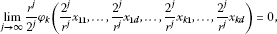(4.1)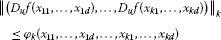(4.2)

for all $u\in U\left(\mathcal{A}\right)$ and ${x}_{11},\dots ,{x}_{1d},\dots ,{x}_{k1},\dots ,{x}_{kd}\in \mathcal{X}$. If there exists an $L<1$ such that

$\begin{array}{c}{\phi }_{k}\left(\stackrel{d}{\stackrel{⏞}{\frac{2}{r}{x}_{11},\frac{2}{r}{x}_{11},\dots ,0}},\dots ,\stackrel{d}{\stackrel{⏞}{\frac{2}{r}{x}_{k1},\frac{2}{r}{x}_{k1},\dots ,0}}\right)\hfill \\ \phantom{\rule{1em}{0ex}}\le \frac{2}{r}L{\phi }_{k}\left(\stackrel{d}{\stackrel{⏞}{{x}_{11},{x}_{11},\dots ,0}},\dots ,\stackrel{d}{\stackrel{⏞}{{x}_{k1},{x}_{k1},\dots ,0}}\right)\hfill \end{array}$

for all $k\in \mathbb{N}$ and ${x}_{11},\dots ,{x}_{k1}\in \mathcal{X}$, then there is a unique $\mathcal{A}$-linear generalized additive mapping $\mathcal{L}:\mathcal{X}\to \mathcal{Y}$ with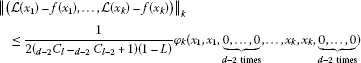(4.3)

for all $k\in \mathbb{N}$ and ${x}_{1},\dots ,{x}_{k}\in \mathcal{X}$.

Proof Put

$X:=\left\{\mathcal{L}:\mathcal{X}\to \mathcal{Y}\right\}$

and

$\begin{array}{rcl}d\left(\mathcal{L},h\right)& =& inf\left\{C\in {\mathbb{R}}_{+}:{\parallel \left(\mathcal{L}\left({x}_{1}\right)-h\left({x}_{1}\right),\dots ,\mathcal{L}\left({x}_{k}\right)-h\left({x}_{k}\right)\right)\parallel }_{k}\\ \le & C{\phi }_{k}\left(\stackrel{d}{\stackrel{⏞}{{x}_{1},{x}_{1},0,\dots ,0}},\dots ,\stackrel{d}{\stackrel{⏞}{{x}_{k},{x}_{k},0,\dots ,0}}\right),k\in \mathbb{N},{x}_{1},\dots ,{x}_{k}\in \mathcal{X}\right\}\end{array}$

for all $\mathcal{L},h\in X$. It is easy to show that $\left(X,d\right)$ is a complete generalized metric space.

Define a mapping $J:X\to X$ by

$J\mathcal{L}\left(x\right):=\frac{r}{2}\mathcal{L}\left(\frac{2}{r}x\right),\phantom{\rule{1em}{0ex}}\mathcal{L}\in X,x\in \mathcal{X}.$

Analysis similar to that in the proof of Theorem 3.1 in  (see also the proof of Lemma 3.2 in ) shows that

$d\left(J\mathcal{L},Jh\right)\le Ld\left(\mathcal{L},h\right),\phantom{\rule{1em}{0ex}}\mathcal{L},h\in X.$

Fix $k\in \mathbb{N}$. Putting $u=1\in U\left(\mathcal{A}\right)$, ${x}_{i1}={x}_{i2}={x}_{1}$, and ${x}_{i3}=\cdots ={x}_{id}=0$ for $i\in \left\{1,\dots ,k\right\}$ in (4.2), we have

$\begin{array}{c}{\parallel \left(rf\left(\frac{2}{r}{x}_{1}\right)-2f\left({x}_{1}\right),\dots ,rf\left(\frac{2}{r}{x}_{k}\right)-2f\left({x}_{k}\right)\right)\parallel }_{k}\hfill \\ \phantom{\rule{1em}{0ex}}\le \frac{1}{{}_{d-2}C_{l}{-}_{d-2}{C}_{l-2}+1}{\phi }_{k}\left({x}_{1},{x}_{1},\underset{d-2\phantom{\rule{0.25em}{0ex}}\mathrm{times}}{\underset{⏞}{0,\dots ,0}},\dots ,{x}_{k},{x}_{k},\underset{d-2\phantom{\rule{0.25em}{0ex}}\mathrm{times}}{\underset{⏞}{0,\dots ,0}}\right),\hfill \end{array}$

because f is odd and $t:{=}_{d-2}{C}_{l}{-}_{d-2}{C}_{l-2}+1{=}_{d-1}{C}_{l}{-}_{d-1}{C}_{l-1}+1$. We thus get

$\begin{array}{c}{\parallel \left(f\left({x}_{1}\right)-\frac{r}{2}f\left(\frac{2}{r}{x}_{1}\right),\dots ,f\left({x}_{k}\right)-\frac{r}{2}f\left(\frac{2}{r}{x}_{k}\right)\right)\parallel }_{k}\hfill \\ \phantom{\rule{1em}{0ex}}\le \frac{1}{2t}{\phi }_{k}\left({x}_{1},{x}_{1},\underset{d-2\phantom{\rule{0.25em}{0ex}}\mathrm{times}}{\underset{⏞}{0,\dots ,0}},\dots ,{x}_{k},{x}_{k},\underset{d-2\phantom{\rule{0.25em}{0ex}}\mathrm{times}}{\underset{⏞}{0,\dots ,0}}\right),\phantom{\rule{1em}{0ex}}{x}_{1},\dots ,{x}_{k}\in \mathcal{X},\hfill \end{array}$

and therefore,

$d\left(f,Jf\right)\le \frac{1}{2t}.$
(4.4)

Consequently, by Theorem 2.2, there exists a mapping $\mathcal{L}:\mathcal{X}\to \mathcal{Y}$ such that

1. (1)

$\mathcal{L}$ is a fixed point of J, i.e.,

$\mathcal{L}\left(\frac{2}{r}x\right)=\frac{2}{r}\mathcal{L}\left(x\right),\phantom{\rule{1em}{0ex}}x\in \mathcal{X},$
(4.5)

and $\mathcal{L}$ is unique in the set

$Y=\left\{\mathcal{L}\in X:d\left(f,\mathcal{L}\right)<\mathrm{\infty }\right\}.$

This means that $\mathcal{L}$ is a unique mapping satisfying (4.5) such that there exists a $C\in \left(0,\mathrm{\infty }\right)$ with

${\parallel \left(\mathcal{L}\left({x}_{1}\right)-f\left({x}_{1}\right),\dots ,\mathcal{L}\left({x}_{k}\right)-f\left({x}_{k}\right)\right)\parallel }_{k}\le C{\phi }_{k}\left({x}_{1},{x}_{1},\underset{d-2\phantom{\rule{0.25em}{0ex}}\mathrm{times}}{\underset{⏞}{0,\dots ,0}},\dots ,{x}_{k},{x}_{k},\underset{d-2\phantom{\rule{0.25em}{0ex}}\mathrm{times}}{\underset{⏞}{0,\dots ,0}}\right)$

for all $k\in \mathbb{N}$ and ${x}_{1},\dots ,{x}_{k}\in \mathcal{X}$.

1. (2)

$d\left({J}^{n}f,\mathcal{L}\right)\to 0$ as $n\to \mathrm{\infty }$. This implies the equality

$\underset{n\to \mathrm{\infty }}{lim}\frac{{r}^{n}}{{2}^{n}}f\left(\frac{{2}^{n}}{{r}^{n}}x\right)=\mathcal{L}\left(x\right)\phantom{\rule{1em}{0ex}}x\in \mathcal{X}.$
(4.6)
2. (3)

$d\left(f,\mathcal{L}\right)\le \frac{1}{1-L}d\left(f,Jf\right)$, which together with (4.4) gives

$d\left(f,\mathcal{L}\right)\le \frac{1}{2t-2tL},$

and therefore, inequality (4.3) holds for all ${x}_{1},\dots ,{x}_{k}\in \mathcal{X}$.

Next, note that the fact that the mapping f is odd and (4.6) imply that $\mathcal{L}$ is odd. Moreover, by (4.1) and (4.2), we get

$\begin{array}{c}{\parallel \left({D}_{1}\mathcal{L}\left({x}_{11},\dots ,{x}_{1d}\right),\dots ,{D}_{1}\mathcal{L}\left({x}_{k1},\dots ,{x}_{kd}\right)\right)\parallel }_{k}\hfill \\ \phantom{\rule{1em}{0ex}}=\underset{n\to \mathrm{\infty }}{lim}\frac{{r}^{n}}{{2}^{n}}{\parallel \left({D}_{1}f\left(\frac{{2}^{n}}{{r}^{n}}{x}_{11},\dots ,\frac{{2}^{n}}{{r}^{n}}{x}_{1d}\right),\dots ,{D}_{1}f\left(\frac{{2}^{n}}{{r}^{n}}{x}_{k1},\dots ,\frac{{2}^{n}}{{r}^{n}}{x}_{kd}\right)\right)\parallel }_{k}\hfill \\ \phantom{\rule{1em}{0ex}}\le \underset{n\to \mathrm{\infty }}{lim}\frac{{r}^{n}}{{2}^{n}}{\phi }_{k}\left(\frac{{2}^{n}}{{r}^{n}}{x}_{11},\dots ,\frac{{2}^{n}}{{r}^{n}}{x}_{1d},\dots ,\frac{{2}^{n}}{{r}^{n}}{x}_{k1},\dots ,\frac{{2}^{n}}{{r}^{n}}{x}_{kd}\right)=0\hfill \end{array}$

for all $k\in \mathbb{N}$ and ${x}_{11},\dots ,{x}_{1d},\dots ,{x}_{k1},\dots ,{x}_{kd}\in \mathcal{X}$, and therefore, $\mathcal{L}$ is a generalized additive mapping.

Fix $u\in U\left(\mathcal{A}\right)$ and $x\in \mathcal{X}$. Using (4.1) and (4.2), we have

$\begin{array}{c}{\parallel \left({D}_{u}\mathcal{L}\left(x,\underset{d-1\phantom{\rule{0.25em}{0ex}}\mathrm{times}}{\underset{⏞}{0,\dots ,0}}\right),\dots ,{D}_{u}\mathcal{L}\left(x,\underset{d-1\phantom{\rule{0.25em}{0ex}}\mathrm{times}}{\underset{⏞}{0,\dots ,0}}\right)\parallel }_{k}\hfill \\ \phantom{\rule{1em}{0ex}}=\underset{n\to \mathrm{\infty }}{lim}\frac{{r}^{n}}{{2}^{n}}{\parallel \left({D}_{u}f\left(\frac{{2}^{n}}{{r}^{n}}x,\underset{d-1\phantom{\rule{0.25em}{0ex}}\mathrm{times}}{\underset{⏞}{0,\dots ,0}}\right),\dots ,{D}_{u}f\left(\frac{{2}^{n}}{{r}^{n}}x,\underset{d-1\phantom{\rule{0.25em}{0ex}}\mathrm{times}}{\underset{⏞}{0,\dots ,0}}\right)\right)\parallel }_{k}\hfill \\ \phantom{\rule{1em}{0ex}}\le \underset{n\to \mathrm{\infty }}{lim}\frac{{r}^{n}}{{2}^{n}}{\phi }_{k}\left(\frac{{2}^{n}}{{r}^{n}}x,\underset{d-1\phantom{\rule{0.25em}{0ex}}\mathrm{times}}{\underset{⏞}{0,\dots ,0}},\dots ,\frac{{2}^{n}}{{r}^{n}}x,\underset{d-1\phantom{\rule{0.25em}{0ex}}\mathrm{times}}{\underset{⏞}{0,\dots ,0}}\right)=0,\hfill \end{array}$

and consequently,

${\left(}_{d-1}{C}_{l}{-}_{d-1}{C}_{l-1}+1\right)r\mathcal{L}\left(\frac{ux}{r}\right)={\left(}_{d-1}{C}_{l}{-}_{d-1}{C}_{l-1}+1\right)u\mathcal{L}\left(x\right).$

Since $\mathcal{L}$ is a generalized additive mapping, from Lemma 4.1 it follows that $\mathcal{L}$ is additive, and therefore,

$\mathcal{L}\left(ux\right)=r\mathcal{L}\left(\frac{ux}{r}\right)=u\mathcal{L}\left(x\right),\phantom{\rule{1em}{0ex}}u\in U\left(\mathcal{A}\right),x\in \mathcal{X}.$

As in the proof of Theorem 3.1, in  one can now show that $\mathcal{L}$ is an $\mathcal{A}$-linear mapping.  □

Corollary 4.4 Let $r\ne 2$ and $\theta ,p\in \left(0,\mathrm{\infty }\right)$. Assume also that $p>1$ for $r>2$, and $p<1$ for $r<2$. If $f:\mathcal{X}\to \mathcal{Y}$ is an odd mapping such that

${\parallel \left({D}_{u}f\left({x}_{11},\dots ,{x}_{1d}\right),\dots ,{D}_{u}f\left({x}_{k1},\dots ,{x}_{kd}\right)\right)\parallel }_{k}\le \theta \left(\sum _{j=1}^{d}{\parallel {x}_{1j}\parallel }^{p}+\cdots +\sum _{j=1}^{d}{\parallel {x}_{kj}\parallel }^{p}\right)$

for all $u\in U\left(\mathcal{A}\right)$, $k\in \mathbb{N}$, and ${x}_{11},\dots ,{x}_{1d},\dots ,{x}_{k1},\dots ,{x}_{kd}\in \mathcal{X}$, then there exists a unique $\mathcal{A}$-linear generalized additive mapping $\mathcal{L}:\mathcal{X}\to \mathcal{Y}$ with

$\begin{array}{c}{\parallel \left(\mathcal{L}\left({x}_{1}\right)-f\left({x}_{1}\right),\dots ,\mathcal{L}\left({x}_{k}\right)-f\left({x}_{k}\right)\right)\parallel }_{k}\hfill \\ \phantom{\rule{1em}{0ex}}\le \frac{{r}^{p-1}\theta }{\left({r}^{p-1}-{2}^{p-1}\right){\left(}_{d-2}{C}_{l}{-}_{d-2}{C}_{l-2}+1\right)}\left({\parallel {x}_{1}\parallel }^{p}+\cdots +{\parallel {x}_{k}\parallel }^{p}\right)\hfill \end{array}$

for all $k\in \mathbb{N}$ and ${x}_{1},\dots ,{x}_{k}\in \mathcal{X}$.

Proof Putting $L=\frac{{2}^{p-1}}{{r}^{p-1}}$ and

$\begin{array}{c}{\phi }_{k}\left({x}_{11},\dots ,{x}_{1d},\dots ,{x}_{k1},\dots ,{x}_{kd}\right)\hfill \\ \phantom{\rule{1em}{0ex}}=\theta \left(\sum _{j=1}^{d}{\parallel {x}_{1j}\parallel }^{p}+\cdots +\sum _{j=1}^{d}{\parallel {x}_{kj}\parallel }^{p}\right),\hfill \end{array}$

for all $k\in \mathbb{N}$ and ${x}_{11},\dots ,{x}_{1d},\dots ,{x}_{k1},\dots ,{x}_{kd}\in \mathcal{X}$, in Theorem 4.3, we get the desired assertion. □

Theorem 4.5 Let $r\ne 2$. Let $f:\mathcal{X}\to \mathcal{Y}$ be an odd mapping for which there is a function $\phi :{\mathcal{X}}^{kd}\to \left[0,\mathrm{\infty }\right)$ such that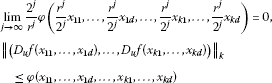(4.7)

for all $u\in U\left(\mathcal{A}\right)$ and all ${x}_{11},\dots ,{x}_{1d},\dots ,{x}_{k1},\dots ,{x}_{kd}\in \mathcal{X}$. If there exists an $L<1$ such that

$\begin{array}{c}\phi \left(\stackrel{d}{\stackrel{⏞}{\frac{r}{2}{x}_{11},\frac{r}{2}{x}_{11},\dots ,0}},\stackrel{d}{\stackrel{⏞}{\frac{r}{2}{x}_{21},\frac{r}{2}{x}_{21},\dots ,0}},\dots ,\stackrel{d}{\stackrel{⏞}{\frac{r}{2}{x}_{k1},\frac{r}{2}{x}_{k1},\dots ,0}}\right)\hfill \\ \phantom{\rule{1em}{0ex}}\le \frac{r}{2}L\phi \left(\stackrel{d}{\stackrel{⏞}{{x}_{11},{x}_{11},\dots ,0}},\stackrel{d}{\stackrel{⏞}{{x}_{21},{x}_{21},\dots ,0}},\dots ,\stackrel{d}{\stackrel{⏞}{{x}_{k1},{x}_{k1},\dots ,0}}\right)\hfill \end{array}$

for all ${x}_{11},{x}_{21},\dots ,{x}_{k1}\in \mathcal{X}$. Then there exists a unique $\mathcal{A}$-linear generalized additive mapping $\mathcal{L}:\mathcal{X}\to \mathcal{Y}$ such that

$\begin{array}{c}\underset{k\in \mathbb{N}}{sup}{\parallel \left(\mathcal{L}\left({x}_{1}\right)-f\left({x}_{1}\right),\dots ,\mathcal{L}\left({x}_{k}\right)-f\left({x}_{k}\right)\right)\parallel }_{k}\hfill \\ \phantom{\rule{1em}{0ex}}\le \underset{k\in \mathbb{N}}{sup}\frac{L}{2{\left(}_{d-2}{C}_{l}{-}_{d-2}{C}_{l-2}+1\right)\left(1-L\right)}\phi \left({x}_{1},{x}_{1},\underset{d-2\phantom{\rule{0.25em}{0ex}}\mathrm{times}}{\underset{⏞}{0,\dots ,0}},\dots ,{x}_{k},{x}_{k},\underset{d-2\phantom{\rule{0.25em}{0ex}}\mathrm{times}}{\underset{⏞}{0,\dots ,0}}\right)\hfill \end{array}$

for all ${x}_{1},\dots ,{x}_{k}\in \mathcal{X}$.

Proof Note that $f\left(0\right)=0$ and $f\left(-x\right)=-f\left(x\right)$ for all $x\in \mathcal{X}$ since f is an odd mapping. Let $u=1\in U\left(\mathcal{A}\right)$. Putting ${x}_{i1}={x}_{i2}={x}_{1}$ and ${x}_{i3}=\cdots ={x}_{im}=0$, $1\le i\le k$ in (4.7), we have

$\begin{array}{c}{\parallel \left(rf\left(\frac{2}{r}{x}_{1}\right)-2f\left({x}_{1}\right),\dots ,rf\left(\frac{2}{r}{x}_{k}\right)-2f\left({x}_{k}\right)\right)\parallel }_{k}\hfill \\ \phantom{\rule{1em}{0ex}}\le \frac{1}{{}_{d-2}C_{l}{-}_{d-2}{C}_{l-2}+1}\phi \left({x}_{1},{x}_{1},\underset{d-2\phantom{\rule{0.25em}{0ex}}\mathrm{times}}{\underset{⏞}{0,\dots ,0}},\dots ,{x}_{k},{x}_{k},\underset{d-2\phantom{\rule{0.25em}{0ex}}\mathrm{times}}{\underset{⏞}{0,\dots ,0}}\right).\hfill \end{array}$

Letting $t:{=}_{d-2}{C}_{l}{-}_{d-2}{C}_{l-2}+1$, we get

$\begin{array}{c}{\parallel \left(f\left({x}_{1}\right)-\frac{2}{r}f\left(\frac{r}{2}{x}_{1}\right),\dots ,f\left({x}_{k}\right)-\frac{2}{r}f\left(\frac{r}{2}{x}_{k}\right)\right)\parallel }_{k}\hfill \\ \phantom{\rule{1em}{0ex}}\le \frac{1}{rt}\phi \left(\frac{r}{2}{x}_{1},\frac{r}{2}{x}_{1},\underset{d-2\phantom{\rule{0.25em}{0ex}}\mathrm{times}}{\underset{⏞}{0,\dots ,0}},\dots ,\frac{r}{2}{x}_{k},\frac{r}{2}{x}_{k},\underset{d-2\phantom{\rule{0.25em}{0ex}}\mathrm{times}}{\underset{⏞}{0,\dots ,0}}\right)\hfill \\ \phantom{\rule{1em}{0ex}}\le \frac{L}{2t}\phi \left({x}_{1},{x}_{1},\underset{d-2\phantom{\rule{0.25em}{0ex}}\mathrm{times}}{\underset{⏞}{0,\dots ,0}},\dots ,{x}_{k},{x}_{k},\underset{d-2\phantom{\rule{0.25em}{0ex}}\mathrm{times}}{\underset{⏞}{0,\dots ,0}}\right)\hfill \end{array}$

for all ${x}_{1},\dots ,{x}_{k}\in \mathcal{X}$.

The rest of the proof is similar to the proof of Theorem 4.3. □

Corollary 4.6 Let $r<2$, and let θ and $p>1$ be positive real numbers, or let $r>2$, and let θ and $p<1$ be positive real numbers. Let $f:\mathcal{X}\to \mathcal{Y}$ be an odd mapping such that

${\parallel \left({D}_{u}f\left({x}_{11},\dots ,{x}_{1d}\right),\dots ,{D}_{u}f\left({x}_{k1},\dots ,{x}_{kd}\right)\right)\parallel }_{k}\le \theta \left(\sum _{j=1}^{d}{\parallel {x}_{1j}\parallel }^{p}+\cdots +\sum _{j=1}^{d}{\parallel {x}_{kj}\parallel }^{p}\right)$

for all $u\in U\left(\mathcal{A}\right)$ and all ${x}_{11},\dots ,{x}_{1d},\dots ,{x}_{k1},\dots ,{x}_{kd}\in \mathcal{X}$. Then there exists a unique $\mathcal{A}$-linear generalized additive mapping $\mathcal{L}:\mathcal{X}\to \mathcal{Y}$ such that

$\begin{array}{c}\underset{k\in \mathbb{N}}{sup}{\parallel \left(\mathcal{L}\left({x}_{1}\right)-f\left({x}_{1}\right),\dots ,\mathcal{L}\left({x}_{k}\right)-f\left({x}_{k}\right)\right)\parallel }_{k}\hfill \\ \phantom{\rule{1em}{0ex}}\le \underset{k\in \mathbb{N}}{sup}\frac{{r}^{p-1}\theta }{\left({2}^{p-1}-{r}^{p-1}\right){\left(}_{d-2}{C}_{l}{-}_{d-2}{C}_{l-2}+1\right)}\left({\parallel {x}_{1}\parallel }^{p}+\cdots +{\parallel {x}_{k}\parallel }^{p}\right)\hfill \end{array}$

for all $x\in X$.

Proof Define

$\phi \left({x}_{11},\dots ,{x}_{1d},\dots ,{x}_{k1},\dots ,{x}_{kd}\right)=\theta \left(\sum _{j=1}^{d}{\parallel {x}_{1j}\parallel }^{p}+\cdots +\sum _{j=1}^{d}{\parallel {x}_{kj}\parallel }^{p}\right).$

Putting $L=\frac{{r}^{p-1}}{{2}^{p-1}}$ in Theorem 4.5, we get the desired result. □

Now we investigate the Hyers-Ulam stability of linear mappings for the case $d=2$.

Theorem 4.7 Let $r\ne 2$. Let $f:\mathcal{X}\to \mathcal{Y}$ be an odd mapping for which there is a function $\phi :{\mathcal{X}}^{2k}\to \left[0,\mathrm{\infty }\right)$ such that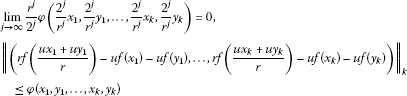(4.8)

for all $u\in U\left(\mathcal{A}\right)$ and all ${x}_{1},\dots {x}_{k},{y}_{1}\dots ,{y}_{k}\in \mathcal{X}$. If there exists an $L<1$ such that

$\phi \left(\frac{2}{r}{x}_{1},\frac{2}{r}{x}_{1},\frac{2}{r}{x}_{2},\frac{2}{r}{x}_{2},\dots ,\frac{2}{r}{x}_{k},\frac{2}{r}{x}_{k}\right)\le \frac{2}{r}L\phi \left({x}_{1},{x}_{1},{x}_{2},{x}_{2},\dots ,{x}_{k},{x}_{k}\right)$

for all ${x}_{1},\dots ,{x}_{k}\in \mathcal{X}$. Then there exists a unique $\mathcal{A}$-linear generalized additive mapping $\mathcal{L}:\mathcal{X}\to \mathcal{Y}$ such that

$\begin{array}{c}\underset{k\in \mathbb{N}}{sup}{\parallel \left(\mathcal{L}\left({x}_{1}\right)-f\left({x}_{1}\right),\dots ,\mathcal{L}\left({x}_{k}\right)-f\left({x}_{k}\right)\right)\parallel }_{k}\hfill \\ \phantom{\rule{1em}{0ex}}\le \underset{k\in \mathbb{N}}{sup}\frac{L}{2\left(1-L\right)}\phi \left({x}_{1},{x}_{1},\dots ,{x}_{k},{x}_{k}\right)\hfill \end{array}$

for all ${x}_{1},\dots ,{x}_{k}\in \mathcal{X}$.

Proof Let $u=1\in U\left(\mathcal{A}\right)$. Putting $x=y$ in (4.8), we have

${\parallel \left(rf\left(\frac{2}{r}{x}_{1}\right)-2f\left({x}_{1}\right),\dots ,rf\left(\frac{2}{r}{x}_{k}\right)-2f\left({x}_{k}\right)\right)\parallel }_{k}\le \phi \left({x}_{1},{x}_{1},\dots ,{x}_{k},{x}_{k}\right)$

for all $x\in X$. So

${\parallel \left(f\left({x}_{1}\right)-\frac{r}{2}f\left(\frac{2}{r}{x}_{1}\right),\dots ,f\left({x}_{k}\right)-\frac{r}{2}f\left(\frac{2}{r}{x}_{k}\right)\right)\parallel }_{k}\le \frac{1}{2}\phi \left({x}_{1},{x}_{1},\dots ,{x}_{k},{x}_{k}\right)$

for all $x\in X$.

The rest of the proof is the same as in the proof of Theorem 4.3. □

Corollary 4.8 Let $r>2$, and let θ and $p>1$ be positive real numbers, or let $r<2$, and let θ and $p<1$ be positive real numbers. Let $f:X\to Y$ be an odd mapping such that

$\begin{array}{c}{\parallel \left(rf\left(\frac{u{x}_{1}+u{y}_{1}}{r}\right)-uf\left({x}_{1}\right)-uf\left({y}_{1}\right),\dots ,rf\left(\frac{u{x}_{k}+u{y}_{k}}{r}\right)-uf\left({x}_{k}\right)-uf\left({y}_{k}\right)\right)\parallel }_{k}\hfill \\ \phantom{\rule{1em}{0ex}}\le \theta \sum _{j=1}^{k}\left({\parallel {x}_{j}\parallel }^{p}+{\parallel {y}_{j}\parallel }^{p}\right)\hfill \end{array}$

for all $u\in U\left(\mathcal{A}\right)$ and for all ${x}_{1},\dots ,{x}_{k}\in \mathcal{X}$. Then there exists a unique $\mathcal{A}$-linear generalized additive mapping $\mathcal{L}:\mathcal{X}\to \mathcal{Y}$ such that

$\underset{k\in \mathbb{N}}{sup}{\parallel \left(\mathcal{L}\left({x}_{1}\right)-f\left({x}_{1}\right),\dots ,\mathcal{L}\left({x}_{k}\right)-f\left({x}_{k}\right)\right)\parallel }_{k}\le \underset{k\in \mathbb{N}}{sup}\frac{{r}^{p-1}\theta }{{r}^{p-1}-{2}^{p-1}}\sum _{j=1}^{k}{\parallel {x}_{j}\parallel }^{p}$

for all ${x}_{1},\dots ,{x}_{k}\in \mathcal{X}$.

Proof Define $\phi \left({x}_{1},{y}_{1},\dots ,{x}_{k},{y}_{k}\right)=\theta {\sum }_{j=1}^{k}\left({\parallel {x}_{j}\parallel }^{p}+{\parallel {y}_{j}\parallel }^{p}\right)$, and apply Theorem 4.7. Then we get the desired result. □

Theorem 4.9 Let $r\ne 2$. Let $f:\mathcal{X}\to \mathcal{Y}$ be an odd mapping for which there is a function $\phi :{\mathcal{X}}^{2k}\to \left[0,\mathrm{\infty }\right)$ such that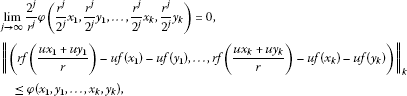(4.9)

for all $u\in U\left(\mathcal{A}\right)$ and all ${x}_{1},\dots ,{x}_{k},{y}_{1},\dots ,{y}_{k}\in \mathcal{X}$. If there exists an $L<1$ such that

$\phi \left(\frac{r}{2}{x}_{1},\frac{r}{2}{x}_{1},\frac{r}{2}{x}_{2},\frac{r}{2}{x}_{2},\dots ,\frac{r}{2}{x}_{k},\frac{r}{2}{x}_{k}\right)\le \frac{r}{2}L\phi \left({x}_{1},{x}_{1},{x}_{2},{x}_{2},\dots ,{x}_{k},{x}_{k}\right)$

for all ${x}_{1},\dots ,{x}_{k}\in \mathcal{X}$. Then there exists a unique $\mathcal{A}$-linear generalized additive mapping $\mathcal{L}:\mathcal{X}\to \mathcal{Y}$ such that

$\underset{k\in \mathbb{N}}{sup}{\parallel \left(\mathcal{L}\left({x}_{1}\right)-f\left({x}_{1}\right),\dots ,\mathcal{L}\left({x}_{k}\right)-f\left({x}_{k}\right)\right)\parallel }_{k}\le \underset{k\in \mathbb{N}}{sup}\frac{1}{2\left(1-L\right)}\phi \left({x}_{1},{x}_{1},\dots ,{x}_{k},{x}_{k}\right)$

for all ${x}_{1},\dots ,{x}_{k}\in \mathcal{X}$.

Proof Let $u=1\in U\left(\mathcal{A}\right)$. Putting $x=y$ in (4.9), we have

${\parallel \left(rf\left(\frac{2}{r}{x}_{1}\right)-2f\left({x}_{1}\right),\dots ,rf\left(\frac{2}{r}{x}_{k}\right)-2f\left({x}_{k}\right)\right)\parallel }_{k}\le \phi \left({x}_{1},{x}_{1},\dots ,{x}_{k},{x}_{k}\right)$

for all ${x}_{1},\dots ,{x}_{k}\in \mathcal{X}$. So

$\begin{array}{rcl}{\parallel \left(f\left({x}_{1}\right)-\frac{2}{r}f\left(\frac{r}{2}{x}_{1}\right),\dots ,f\left({x}_{k}\right)-\frac{2}{r}f\left(\frac{r}{2}{x}_{k}\right)\right)\parallel }_{k}& \le & \frac{1}{r}\phi \left(\frac{r}{2}{x}_{1},\frac{r}{2}{x}_{1},\dots ,\frac{r}{2}{x}_{k},\frac{r}{2}{x}_{k}\right)\\ \le & \frac{1}{2}L\phi \left({x}_{1},{x}_{1},\dots ,{x}_{k},{x}_{k}\right)\end{array}$

for all ${x}_{1},\dots ,{x}_{k}\in \mathcal{X}$.

The rest of the proof is similar to the proof of Theorem 4.3. □

Corollary 4.10 Let $r>2$, and let θ and $p>1$ be positive real numbers. Or let $r<2$, and let θ and $p<1$ be positive real numbers. Let $f:X\to Y$ be an odd mapping such that

$\begin{array}{c}{\parallel \left(rf\left(\frac{u{x}_{1}+u{y}_{1}}{r}\right)-uf\left({x}_{1}\right)-uf\left({y}_{1}\right),\dots ,rf\left(\frac{u{x}_{k}+u{y}_{k}}{r}\right)-uf\left({x}_{k}\right)-uf\left({y}_{k}\right)\right)\parallel }_{k}\hfill \\ \phantom{\rule{1em}{0ex}}\le \theta \sum _{j=1}^{k}\left({\parallel {x}_{j}\parallel }^{p}+{\parallel {y}_{j}\parallel }^{p}\right)\hfill \end{array}$

for all $u\in U\left(\mathcal{A}\right)$ and all ${x}_{1},\dots ,{x}_{k}\in \mathcal{X}$. Then there exists a unique $\mathcal{A}$-linear generalized additive mapping $\mathcal{L}:\mathcal{X}\to \mathcal{Y}$ such that

$\underset{k\in \mathbb{N}}{sup}{\parallel \left(\mathcal{L}\left({x}_{1}\right)-f\left({x}_{1}\right),\dots ,\mathcal{L}\left({x}_{k}\right)-f\left({x}_{k}\right)\right)\parallel }_{k}\le \underset{k\in \mathbb{N}}{sup}\frac{{r}^{p-1}\theta }{{2}^{p-1}-{r}^{p-1}}\sum _{j=1}^{k}{\parallel {x}_{j}\parallel }^{p}$

for all ${x}_{1},\dots ,{x}_{k}\in \mathcal{X}$.

Proof Define $\phi \left({x}_{1},{y}_{1},\dots ,{x}_{k},{y}_{k}\right)=\theta {\sum }_{j=1}^{k}\left({\parallel {x}_{j}\parallel }^{p}+{\parallel {y}_{j}\parallel }^{p}\right)$, and apply Theorem 4.9. Then we get the desired result. □

## 5 Isomorphisms in unital multi-${C}^{\ast }$-algebras

Throughout this section, assume that $\mathcal{A}$ and $\mathcal{B}$ are unital multi-${C}^{\ast }$-algebras with unit e. Let $U\left(\mathcal{A}\right)$ be the set of unitary elements in $\mathcal{A}$.

We investigate ${C}^{\ast }$-algebra isomorphisms in unital multi-${C}^{\ast }$-algebras.

Theorem 5.1 Let $r\ne 2$. Let $h:\mathcal{A}\to \mathcal{B}$ be an odd bijective mapping satisfying $h\left(\frac{{2}^{n}}{{r}^{n}}uy\right)=h\left(\frac{{2}^{n}}{{r}^{n}}u\right)h\left(y\right)$ for all $u\in U\left(\mathcal{A}\right)$, all $y\in \mathcal{A}$, and $n=0,1,2,\dots$, for which there exists a function $\phi :{\mathcal{A}}^{kd}\to \left[0,\mathrm{\infty }\right)$ such that

$\begin{array}{c}\underset{j\to \mathrm{\infty }}{lim}\frac{{r}^{j}}{{2}^{j}}\phi \left(\frac{{2}^{j}}{{r}^{j}}{x}_{11},\dots ,\frac{{2}^{j}}{{r}^{j}}{x}_{1d},\dots ,\frac{{2}^{j}}{{r}^{j}}{x}_{k1},\dots ,\frac{{2}^{j}}{{r}^{j}}{x}_{kd}\right)=0,\hfill \\ {\parallel \left({D}_{\mu }h\left({x}_{11},\dots ,{x}_{1d}\right),\dots ,{D}_{\mu }h\left({x}_{k1},\dots ,{x}_{kd}\right)\right)\parallel }_{k}\hfill \\ \phantom{\rule{1em}{0ex}}\le \phi \left({x}_{11},\dots ,{x}_{1d},\dots ,{x}_{k1},\dots ,{x}_{kd}\right),\hfill \\ {\parallel \left(h\left(\frac{{2}^{n}}{{r}^{n}}{u}_{1}^{\ast }\right)-h{\left(\frac{{2}^{n}}{{r}^{n}}{u}_{1}\right)}^{\ast },\dots ,h\left(\frac{{2}^{n}}{{r}^{n}}{u}_{k}^{\ast }\right)-h{\left(\frac{{2}^{n}}{{r}^{n}}{u}_{k}\right)}^{\ast }\right)\parallel }_{k}\hfill \\ \phantom{\rule{1em}{0ex}}\le \phi \left(\underset{d\phantom{\rule{0.25em}{0ex}}\mathrm{times}}{\underset{⏞}{\frac{{2}^{n}}{{r}^{n}}{u}_{1},\dots ,\frac{{2}^{n}}{{r}^{n}}{u}_{1}}},\dots ,\underset{d\phantom{\rule{0.25em}{0ex}}\mathrm{times}}{\underset{⏞}{\frac{{2}^{n}}{{r}^{n}}{u}_{k},\dots ,\frac{{2}^{n}}{{r}^{n}}{u}_{k}}}\right)\hfill \end{array}$

for all $\mu \in {S}^{1}:=\left\{\lambda \in \mathbb{C}\mid |\lambda |=1\right\}$, all ${u}_{1},\dots ,{u}_{k}\in U\left(\mathcal{A}\right)$, $n=0,1,2,\dots$, and all ${x}_{11},\dots ,{x}_{kd}\in A$. Assume that ${lim}_{n\to \mathrm{\infty }}\frac{{r}^{n}}{{2}^{n}}h\left(\frac{{2}^{n}}{{r}^{n}}e\right)$ is invertible. Then the odd bijective mapping $h:\mathcal{A}\to \mathcal{B}$ is a ${C}^{\ast }$-algebra isomorphism.

Proof Consider the multi-${C}^{\ast }$-algebras $\mathcal{A}$ and $\mathcal{B}$ as left Banach modules over the unital multi-${C}^{\ast }$-algebra $\mathbb{C}$. By Theorem 4.3, there exists a unique $\mathbb{C}$-linear generalized additive mapping $\mathcal{H}:\mathcal{A}\to \mathcal{B}$ such that

$\begin{array}{c}\underset{k\in \mathbb{N}}{sup}{\parallel \left(h\left({x}_{1}\right)-\mathcal{H}\left({x}_{1}\right),\dots ,h\left({x}_{k}\right)-\mathcal{H}\left({x}_{k}\right)\parallel }_{k}\hfill \\ \phantom{\rule{1em}{0ex}}\le \underset{k\in \mathbb{N}}{sup}\frac{1}{2{\left(}_{d-2}{C}_{l}{-}_{d-2}{C}_{l-2}+1\right)}\phi \left({x}_{1},{x}_{1},\underset{d-2\phantom{\rule{0.25em}{0ex}}\mathrm{times}}{\underset{⏞}{0,\dots ,0}},\dots ,{x}_{k},{x}_{k},\underset{d-2\phantom{\rule{0.25em}{0ex}}\mathrm{times}}{\underset{⏞}{0,\dots ,0}}\right)\hfill \end{array}$

for all ${x}_{1},\dots ,{x}_{k}\in \mathcal{A}$ in which $\mathcal{H}:\mathcal{A}\to \mathcal{B}$ is given by

$\mathcal{H}\left(x\right)=\underset{n\to \mathrm{\infty }}{lim}\frac{{r}^{n}}{{2}^{n}}h\left(\frac{{2}^{n}}{{r}^{n}}x\right)$

for all $x\in \mathcal{A}$.

The rest of the proof is similar to the proof of Theorem 4.1 of . □

Corollary 5.2 Let $r>2$, and let θ and $p>1$ be positive real numbers. Or let $r<2$, and let θ and $p<1$ be positive real numbers. Let $h:\mathcal{A}\to \mathcal{B}$ be an odd bijective mapping satisfying $h\left(\frac{{2}^{n}}{{r}^{n}}uy\right)=h\left(\frac{{2}^{n}}{{r}^{n}}u\right)h\left(y\right)$ for all $u\in U\left(\mathcal{A}\right)$, all $y\in \mathcal{A}$, and all $n=0,1,2,\dots$, such that

$\begin{array}{c}{\parallel \left({D}_{\mu }h\left({x}_{11},\dots ,{x}_{1d}\right),\dots ,{D}_{\mu }h\left({x}_{k1},\dots ,{x}_{kd}\right)\right)\parallel }_{k}\le \theta \sum _{j=1}^{d}\left({\parallel {x}_{1j}\parallel }^{p}+\cdots +{\parallel {x}_{kj}\parallel }^{p}\right),\hfill \\ {\parallel \left(h\left(\frac{{2}^{n}}{{r}^{n}}{u}_{1}^{\ast }\right)-h{\left(\frac{{2}^{n}}{{r}^{n}}{u}_{1}\right)}^{\ast },\dots ,h\left(\frac{{2}^{n}}{{r}^{n}}{u}_{k}^{\ast }\right)-h{\left(\frac{{2}^{n}}{{r}^{n}}{u}_{k}\right)}^{\ast }\right)\parallel }_{k}\le kd\frac{{2}^{pn}}{{r}^{pn}}\theta \hfill \end{array}$

for all $\mu \in {S}^{1}$, all $u\in U\left(\mathcal{A}\right)$, $n=0,1,2,\dots$, and all ${x}_{11},\dots ,{x}_{kd}\in \mathcal{A}$. Assume that ${lim}_{n\to \mathrm{\infty }}\frac{{r}^{n}}{{2}^{n}}h\left(\frac{{2}^{n}}{{r}^{n}}e\right)$ is invertible. Then the odd bijective mapping $h:\mathcal{A}\to \mathcal{B}$ is a ${C}^{\ast }$-algebra isomorphism.

Proof Define $\phi \left({x}_{11},\dots ,{x}_{1d},\dots ,{x}_{k1},\dots ,{x}_{kd}\right)=\theta {\sum }_{j=1}^{d}\left({\parallel {x}_{1j}\parallel }^{p}+\cdots +{\parallel {x}_{kj}\parallel }^{p}\right)$, and apply Theorem 5.1. Then we get the desired result. □

## References

1. Rassias TM: On the stability of the linear mapping in Banach spaces. Proc. Am. Math. Soc. 1978, 72: 297–300. 10.1090/S0002-9939-1978-0507327-1

2. Găvruta P: A generalization of the Hyers-Ulam-Rassias stability of approximately additive mappings. J. Math. Anal. Appl. 1994, 184: 431–436. 10.1006/jmaa.1994.1211

3. Park C: On the stability of the linear mapping in Banach modules. J. Math. Anal. Appl. 2002, 275: 711–720. 10.1016/S0022-247X(02)00386-4

4. Rassias TM: On the stability of functional equations and a problem of Ulam. Acta Appl. Math. 2000, 62: 23–130. 10.1023/A:1006499223572

5. Eshaghi Gordji M, Khodaei H: Stability of Functional Equations. Lap Lambert Academic Publishing, Saarbrücken; 2010.

6. Jung S-M Springer Optimization and Its Applications 48. In Hyers-Ulam-Rassias Stability of Functional Equations in Nonlinear Analysis. Springer, New York; 2011.

7. Baak C, Boo D, Rassias TM:Generalized additive mapping in Banach modules and isomorphisms between ${C}^{\ast }$-algebras. J. Math. Anal. Appl. 2006, 314: 150–156. 10.1016/j.jmaa.2005.03.099

8. Cădariu L, Radu V: Fixed points and the stability of Jensen’s functional equation. J. Inequal. Pure Appl. Math. 2003., 4: Article ID 4

9. Radu V: The fixed point alternative and the stability of functional equations. Fixed Point Theory 2003, 4: 91–96.

10. Diaz J, Margolis B: A fixed point theorem of the alternative for contractions on a generalized complete metric space. Bull. Am. Math. Soc. 1968, 74: 305–309. 10.1090/S0002-9904-1968-11933-0

11. Dales, HG, Polyakov, ME: Multi-normed spaces and multi-Banach algebras. Preprint

12. Dales HG, Moslehian MS: Stability of mappings on multi-normed spaces. Glasg. Math. J. 2007, 49: 321–332. 10.1017/S0017089507003552

13. Moslehian MS, Nikodem K, Popa D: Asymptotic aspect of the quadratic functional equation in multi-normed spaces. J. Math. Anal. Appl. 2009, 355: 717–724. 10.1016/j.jmaa.2009.02.017

14. Moslehian MS: Superstability of higher derivations in multi-Banach algebras. Tamsui Oxf. J. Math. Sci. 2008, 24: 417–427.

15. Dales HG London Mathematical Society Monographs. New Series 24. In Banach Algebras and Automatic Continuity. Oxford University Press, Oxford; 2000.

## Acknowledgements

The authors are grateful to the reviewers for their valuable comments and suggestions.

## Author information

Authors

### Competing interests

The authors declare that they have no competing interests.

### Authors’ contributions

All authors carried out the proof. All authors conceived of the study and participated in its design and coordination. All authors read and approved the final manuscript.

## Rights and permissions

Reprints and Permissions

Park, C., Saadati, R. Approximation of a generalized additive mapping in multi-Banach modules and isomorphisms in multi-${C}^{\ast }$-algebras: a fixed-point approach. Adv Differ Equ 2012, 162 (2012). https://doi.org/10.1186/1687-1847-2012-162

• Accepted:

• Published:

• DOI: https://doi.org/10.1186/1687-1847-2012-162

### Keywords

• ${C}^{\ast }$-algebra isomorphism
• fixed point
• multi-Banach module over multi-${C}^{\ast }$-algebra